## Example Questions

### Example Question #232 : Exponents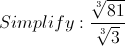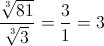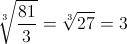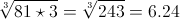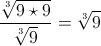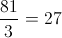Explanation: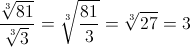### Example Question #231 : Exponents

Rationalize the denominator: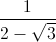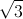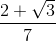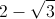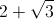Explanation:

The conjugate ofis.

Now multiply both the numerator and the denominator byand you get: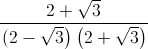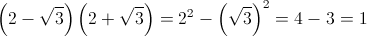Hence we get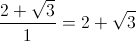### Example Question #234 : Exponents

Solve for: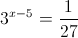Explanation: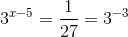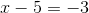### Example Question #235 : Exponents

Solve for.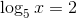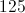Explanation: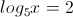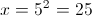### Example Question #236 : Exponents

If,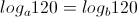What does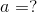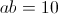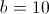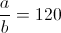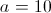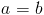Explanation:

If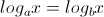,

then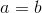.

### Example Question #237 : Exponents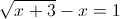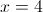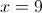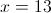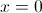Explanation:

From the equation in the problem statement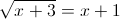Now squaring both sides we get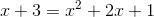this is a quadratic equation which equals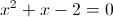and the factors of this equation are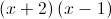This gives us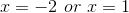.

However, if we plug these solutions back into the original equation,does not create an equality. Therefore,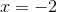is an extraneous solution.